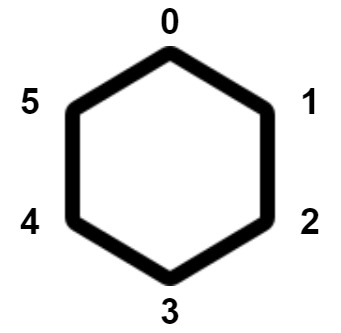2550. Count Collisions of Monkeys on a Polygon
Medium
138
431

There is a regular convex polygon with `n` vertices. The vertices are labeled from `0` to `n - 1` in a clockwise direction, and each vertex has exactly one monkey. The following figure shows a convex polygon of `6` vertices.Each monkey moves simultaneously to a neighboring vertex. A neighboring vertex for a vertex `i` can be:

• the vertex `(i + 1) % n` in the clockwise direction, or
• the vertex `(i - 1 + n) % n` in the counter-clockwise direction.

A collision happens if at least two monkeys reside on the same vertex after the movement or intersect on an edge.

Return the number of ways the monkeys can move so that at least one collision happens. Since the answer may be very large, return it modulo `109 + 7`.

Note that each monkey can only move once.

Example 1:

```Input: n = 3
Output: 6
Explanation: There are 8 total possible movements.
Two ways such that they collide at some point are:
- Monkey 1 moves in a clockwise direction; monkey 2 moves in an anticlockwise direction; monkey 3 moves in a clockwise direction. Monkeys 1 and 2 collide.
- Monkey 1 moves in an anticlockwise direction; monkey 2 moves in an anticlockwise direction; monkey 3 moves in a clockwise direction. Monkeys 1 and 3 collide.
It can be shown 6 total movements result in a collision.
```

Example 2:

```Input: n = 4
Output: 14
Explanation: It can be shown that there are 14 ways for the monkeys to collide.
```

Constraints:

• `3 <= n <= 109`
Accepted
14.7K
Submissions
55.5K
Acceptance Rate
26.5%

Seen this question in a real interview before?
1/4
Yes
No

Discussion (0)

Related Topics